# Application of Derivatives Class 12 Notes Mathematics

Application of Derivatives class 12 Notes Mathematics in PDF are available for free download in myCBSEguide mobile app. The best app for CBSE students now provides Application of Derivatives class 12 Notes latest chapter wise notes for quick preparation of CBSE board exams and school-based annual examinations. Class 12 Mathematics notes on chapter 6 Application of Derivatives are also available for download in CBSE Guide website.

## Application of Derivatives Class 12 Notes Mathematics

Download CBSE class 12th revision notes for chapter 6 Application of Derivatives in PDF format for free. Download revision notes for Application of Derivatives class 12 Notes and score high in exams. These are the Application of Derivatives class 12 Notes prepared by team of expert teachers. The revision notes help you revise the whole chapter 6 in minutes. Revision notes in exam days is one of the best tips recommended by teachers during exam days.

## CBSE Class 12 Maths Revision Notes Chapter 6 Application of Derivatives

• If a quantity y varies with another quantity x, satisfying some rule y = f(x), then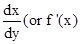represents the rate of change of y with respect to x and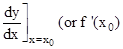represents the rate of change of y with respect to x at x = xo.
• If two variables x and y are varying with respect to another variable t, i.e., if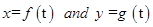then by Chain Rule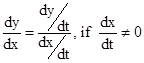• A function f is said to be increasing on an interval (a,  b) if  in  for all   Alternatively, if   for each  in, then f(x) is an increasing funciton on (a, b).
• A function f is said to be decreasing on an interval (a,  b) if  in  for all   Alternatively, if   for each  in, then f(x) is an decreasing funciton on (a, b).
• The equation of the tangent at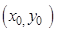to the curve y =  f (x) is given by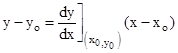• If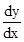does not exist at the point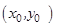, then the tangent at this point is parallel to the y-axis and its equation is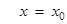.
• If tangent to a curve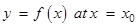is parallel to x-axis, then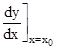= 0
• Equation of the normal to the curve y = f (x) at a point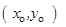is given by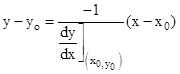• If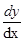at the pointis zero, then equation of the normal is .
• If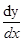at the pointdoes not exist, then the normal is parallel to x-axis and its equation is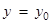.
• Let y = f (x), ∆x be a small increment in x and ∆y be the increment in y corresponding to the increment in x, i.e., ∆y = f (x + ∆x) –  f (x). Then  given by  or  is a good appApplication of Derivatives Class 12 Notes Mathematicsroximation of ∆y when  dx x = ∆  is relatively small and we denote it by dy ≈ ∆y.
• A point c in the domain of a function f at which either f ′(c) = 0 or f is not differentiable is called a critical point of f.
• First Derivative Test : Let f  be a function defined on an open interval I. Let f   be continuous at a critical point c in I. Then,

(i) If f ′(x) changes sign from positive to negative as x increases through c, i.e., if f ′(x) > 0 at every point sufficiently close to and to the left of c, and f ′(x) < 0 at every point sufficiently close to and to the right of c, then c is a point of local maxima.

(ii) If f ′(x) changes sign from negative to positive as x increases through c, i.e., if f ′(x) < 0 at every point sufficiently close to and to the left of c, and f ′(x) > 0 at every point sufficiently close to and to the right of c, then c is a point of local minima.

(iii) If f ′(x) does not change sign as x increases through c, then c is neither a point of local maxima nor a point of local minima. In fact, such a point is called point of inflexion.

• Second Derivative Test: Let f be a function defined on an interval I and c ∈ I. Let f   be twice differentiable at c. Then,

(i) x = c is a point of local maxima if f ′(c) = 0 and f ″(c) < 0
The values f (c) is local maximum value of   f.

(ii) x = c is a point of local minima if f ′(c) = 0 and f ″(c) > 0
In this case, f (c) is local minimum value of f.

(iii) The test fails if f ′(c) = 0 and f ″(c) = 0.
In this case, we go back to the first derivative test and find whether c is a point of maxima, minima or a point of inflexion.

• Working rule for finding absolute maxima and/or absolute minima

Step 1: Find all critical points of f in the interval, i.e., find points x where either f ′(x) = 0 or f is not differentiable.

Step 2: Take the end points of the interval.

Step 3: At all these points (listed in Step 1 and 2), calculate the values of f.

Step 4: Identify the maximum and minimum values of f out of the values calculated in Step 3.

This maximum value will be the absolute maximum value of f and the minimum value will be the absolute minimum value of f .

## CBSE Class 12 Revision Notes and Key Points

Application of Derivatives class 12 Notes Mathematics. CBSE quick revision note for class-12 Chemistry Physics Math’s, Biology and other subject are very helpful to revise the whole syllabus during exam days. The revision notes covers all important formulas and concepts given in the chapter. Even if you wish to have an overview of a chapter, quick revision notes are here to do if for you. These notes will certainly save your time during stressful exam days.

To download Application of Derivatives class 12 Notes Mathematics, sample paper for class 12 Physics, Chemistry, Biology, History, Political Science, Economics, Geography, Computer Science, Home Science, Accountancy, Business Studies and Home Science; do check myCBSEguide app or website. myCBSEguide provides sample papers with solution, test papers for chapter-wise practice, NCERT Application of Derivatives, NCERT Exemplar Application of Derivatives, quick revision notes for ready reference, CBSE guess papers and CBSE important question papers. Sample Paper all are made available through the best app for CBSE students and myCBSEguide website.Test Generator

Create question paper PDF and online tests with your own name & logo in minutes.myCBSEguide

Question Bank, Mock Tests, Exam Papers, NCERT Solutions, Sample Papers, Notes